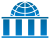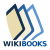# Trigonometric substitutionWikiversity has learning materials about Trigonometric SubstitutionsWikibooks has a book on the topic of: Calculus/Integration techniques/Trigonometric Substitution

In mathematics, Trigonometric substitution is the substitution of trigonometric functions for other expressions. One may use the trigonometric identities to simplify certain integrals containing radical expressions:

Substitution 1. If the integrand contains a2  x2, letand use the identitySubstitution 2. If the integrand contains a2 + x2, letand use the identitySubstitution 3. If the integrand contains x2  a2, letand use the identity## Examples

### Integrals containing a2 − x2

In the integralwe may useNote that the above step requires that a > 0 and cos(θ) > 0; we can choose the a to be the positive square root of a2; and we impose the restriction on θ to be −π/2 < θ < π/2 by using the arcsin function.

For a definite integral, one must figure out how the bounds of integration change. For example, as x goes from 0 to a/2, then sin(θ) goes from 0 to 1/2, so θ goes from 0 to π/6. Then we haveSome care is needed when picking the bounds. The integration above requires that −π/2 < θ < π/2, so θ going from 0 to π/6 is the only choice. If we had missed this restriction, we might have picked θ to go from π to 5π/6, which would give us the negative of the result.

### Integrals containing a2 + x2

In the integralwe may writeso that the integral becomes(provided a  0).

### Integrals containing x2 − a2

Integrals likeshould be done by partial fractions rather than trigonometric substitutions. However, the integralcan be done by substitution:We can then solve this using the formula for the integral of secant cubed.

## Substitutions that eliminate trigonometric functions

Substitution can be used to remove trigonometric functions. In particular, see Tangent half-angle substitution.

For instance,## Hyperbolic substitution

Substitutions of hyperbolic functions can also be used to simplify integrals.

In the integral, make the substitution,.

Then, using the identitiesand,